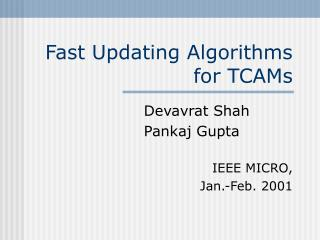# Fast Updating Algorithms for TCAMs - PowerPoint PPT PresentationDownload PresentationFast Updating Algorithms for TCAMs

Presentation Description
Download Presentation## Fast Updating Algorithms for TCAMs

- - - - - - - - - - - - - - - - - - - - - - - - - - - E N D - - - - - - - - - - - - - - - - - - - - - - - - - - -
##### Presentation Transcript

1. Fast Updating Algorithms for TCAMs Devavrat Shah Pankaj Gupta IEEE MICRO, Jan.-Feb. 2001

2. Outline • Background • Introduction to TCAMs • Provided algorithms • Simulation • Conclusion and discussion

3. Background • A collection of rules is called a policy database or a classifier • Packet classification • To find a best matching rule • Identification of the flow • Generalization of the routing lookup • Routers must keep pace with link speeds

4. Intro. to TCAMs • Fully associative memory • Parallel search for every element in the array • Take three logic states • 0, 1, or don’t-care X • Store each field as a (var, mask) pair • (10000, 11000) represents the 5-bit prefix 10* • Store forwarding table entries in order of decreasing prefix lengths (priorities) • The lower address has higher priority

5. Intro. to TCAMs (cont’d) • Increasingly being deployed • Simplicity and speed (10 ns per clock) • Forwarding table updates complicate keeping the list of prefixes in sorted order

6. Intro. to TCAMs (cont’d)

7. Intro. to TCAMs (cont’d) • If a new prefix 103.23.128/18 is added to the table • There’s no empty space between prefixes P1 and P2! • There are two intuitive solutions below.

8. Intuitive solution 1 • Shift prefixes P2 to P5 downward by one location each • This costs O(N), where N is the # of prefixes

9. Intuitive solution 2 • Keeps a few empty memory locations at all X nonempty ones • Degenerates to O(N) if empty space is filled up • Wastes precious CAM space

10. Provided algorithm 1 • Two prefixes of the same length don’t need to be ordered • Prefix-length ordering constraint • Referred to here as L-algorithm, which can be optimized to L/2

11. Provided algorithm 1 (cont’d) • Keep all the unused entries in the center • Swap at most L/2 entries to obtain an unused entry • The arrangement of Fig. 4 can again improve the time

12. Provided algorithm 2 • PLO constraint is more restrictive • The constraint can be relaxed to only overlapping prefixes • Prefixes that lie on the same chain of the trie need to be ordered • Chain-ancestor ordering constraint

13. Provided algorithm 2 (cont’d) • There’s an ordering constraint between pi and pj iff one is a prefix of the other • ex. 103.23.3/24 and 103.23/16 • Decrease the update to D/2, where D is the max length of any chain in the trie

14. Provided algorithm 2 (cont’d) • Terminology • LC(p) • len(LC(p)) • rootpath(p) • ancestor of p • prefix-child of p • hcld(p) • HCN(p)

15. Insertion • Case I. Assume q is to be inserted above the pool • One empty entry can be created by moving prefixes on LC(q) downward one by one, starting from pj to the border of the pool • The movement is less than D/2, where D is len(LC(q))

16. Insertion (cont’d) • Case II. Assume q is to be inserted below the pool • One empty entry can be created by moving prefixes on HCN(q) upward one by one, starting from pj to the border of the pool • Again, the movement is less than D/2 pj pi Pi + 1

17. Deletion • Two exceptions compared with insertion • It works in reverse • It works on the chain that has prefix p adjacent to the free space pool • The new unused entry is rippled by moving prefixes downward on the chain

18. 4 2 3 1 2 2 1 1 Auxiliary trie data structure • To determine LC(p) and HCN(p) quickly, the following additional fields in every trie node are maintained • wt(p): the weight of each node p • wt_ptr(p): the child with the highest weight • hcld_ptr(p): the child at the highest location The weight of each node

19. Simulation

20. Simulation (cont’d)

21. Simulation (cont’d)

22. Simulation (cont’d)

23. Simulation (cont’d)

24. Conclusion and discussion • The PLO_OPT algorithm improves update speed by a factor of two over the best-known solution • The CAO_OPT algorithm completes one prefix update in slightly greater than one memory movement per update operation • Some disadvantages of TCAMs • More prices, more power consumption, less capacity, dealing with prefixes only